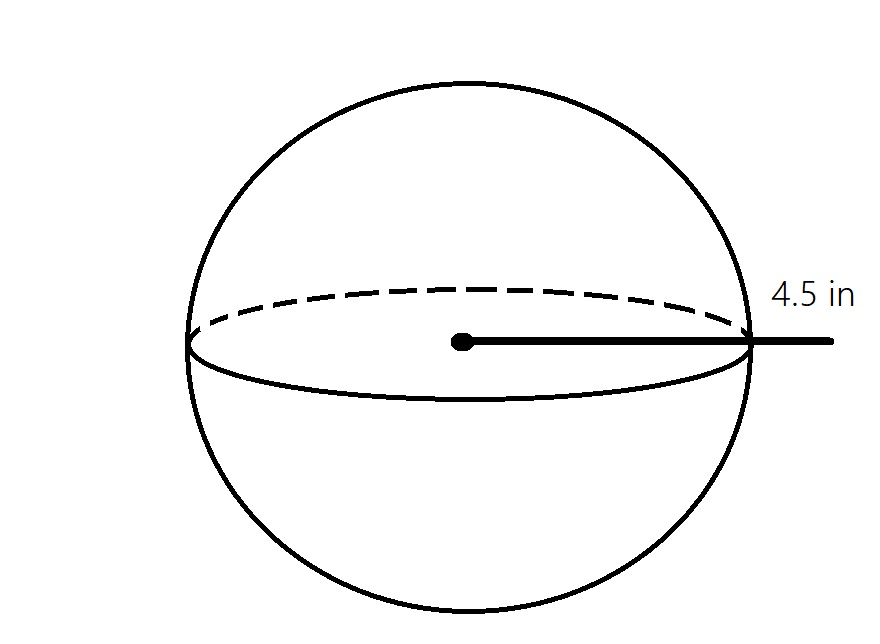Determine how much air could fit inside a soccer ballkloseyq 2021-12-19 Answered
Determine how much air could fit inside a soccer ball with radius of 4.5 inches. Use 3.14 for $$\displaystyle\pi$$• Questions are typically answered in as fast as 30 minutes

Solve your problem for the price of one coffee

• Math expert for every subject
• Pay only if we can solve itAlex Sheppard

Step 1
That mean we have to find the volume of the Soccer ball.
Since it is a Shere with radius
$$\displaystyle{r}={4.5}\ \text{inches}$$
$$\displaystyle\therefore$$ volume of Soccer ball $$=\frac{4}{3}\barwedge r^{3}$$
$$\displaystyle{V}={\frac{{{4}}}{{{3}}}}\times\overline{\wedge}\times{\left({4.5}\right)}^{{{3}}}$$
$$\displaystyle={\frac{{{4}}}{{{3}}}}\times\overline{\wedge}\times{91.128}$$
$$\displaystyle={\frac{{{4}}}{{{3}}}}\times{3.14}\times{91.725}$$
$$\displaystyle={\frac{{{4}\times{286.1325}}}{{{3}}}}$$
$$\displaystyle={\frac{{{1144.53}}}{{{3}}}}$$
$$\displaystyle={381.51}\ in^{{{3}}}$$
$$\displaystyle\therefore{V}={381.51}\ in^{{{3}}}$$

Not exactly what you’re looking for?poleglit3
Step 1
The formula for a sphere is
$$\displaystyle{V}={\frac{{{4}}}{{{3}}}}{\left({3.14}\right\rbrace}{\left({4.5}^{{{3}}}\right)}$$
When you calculate that, you get.
$$\displaystyle{\frac{{{243}{\left({3.14}\right)}}}{{{2}}}}$$
Now you divide by 2.
$$\displaystyle={381.7}$$